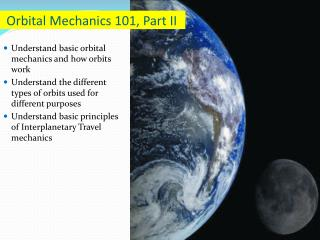# Orbital Mechanics 101, Part II - PowerPoint PPT PresentationDownload PresentationOrbital Mechanics 101, Part II

Orbital Mechanics 101, Part IIDownload Presentation## Orbital Mechanics 101, Part II

- - - - - - - - - - - - - - - - - - - - - - - - - - - E N D - - - - - - - - - - - - - - - - - - - - - - - - - - -
##### Presentation Transcript

1. Orbital Mechanics 101, Part II • Understand basic orbital mechanics and how orbits work • Understand the different types of orbits used for different purposes • Understand basic principles of Interplanetary Travel mechanics

2. Spheres of Influence • A body’s sphere of influence (SOI) is the surrounding volume in which its gravity dominates a spacecraft. • In theory, SOI is infinite. • In practice, as a spacecraft gets farther away, another body’s gravity dominates. • The size of a planet’s SOI depends on: • The planet’s mass • How close the planet is to the Sun (Sun’s gravity overpowers that of closer planets)

3. Types of Orbits • Low-Earth orbit – an orbit up to about 1,240 miles above the Earth • Medium-Earth orbit– one with an altitude of about 12,400 miles • High-Earth orbit – an orbit at an altitude of about 22,300 miles

4. Maneuvering in Space • Orbit Changes • Moving From One Orbit to Another • Using the Hohmann Transfer • Rendezvous in Space • Gravity Assist Velocity

5. Simple Orbit Changes:Moving from one orbit to another • Moving from one orbit to another is a complex and orchestrated dance of speed and fuel consumption • When we maneuver in space, we need to factor fuel into the process. • Fuel is EVERYTHING in Space First space rendezvous of two spacecraft (Gemini 6 and 7) in December 1965

6. Simple Orbit Changes:Moving from one orbit to another • In 1925 a German engineer, Walter Hohmann, thought of a fuel-efficient way to transfer spacecraft between orbits—the Hohmann Transfer. • The Hohmann Transfer uses an elliptical transfer orbit tangent to the initial and final orbits.

7. Simple Orbit Changes:Using the Hohmann Transfer • A spacecraft changes its energy by increasing or decreasing its velocity — in essence firing rocket engines. • Adding or subtracting velocity changes the orbit’s energy

8. Hohmann Transfer (Application to Rendezvous) First space rendezvous of two spacecraft (Gemini 6 and 7) in December 1965

9. Interplanetary Travel • Four bodies are involved: • Sun • Departure Planet • Target Planet • Spacecraft • Analyzing the gravitational forces (Remember that Newton guy and his Law of Universal Gravitation) from all three central bodies on the spacecraft would amount to a “four-body” problem. Far to complicated, so…. • Simplify (A process called “Patched Conic”Approximation) • Break into familiar, manageable pieces. • Consider as three two-body problems.

10. “Patched Conic” Approximation

11. Gravity-Assist Trajectories • Process using a planet’s gravitational field and orbital velocity to “sling shot” a spacecraft, changing its velocity (in magnitude and direction) with respect to the Sun.

12. Gravity-Assist Trajectories • As a spacecraft enters a planet’s sphere of influence (SOI), it coasts on a hyperbolic trajectory around the planet. • Then, the planet pulls it in the direction of the planet’s motion, increasing (or decreasing) its velocity relative to the Sun.

13. Gravity-Assist Trajectories Generally, gravity-assisted trajectories consist of two types: • A gravity assist that changes the magnitude of a spacecraft’s velocity is called orbit pumping. • Using a planet’s gravity to change the direction of travel is called orbit cranking.

14. Gravity-Assist Trajectories Gravity Launch Game http://www.sciencenetlinks.com/interactives/gravity.html

15. Orbital Mechanics 101, Part II • Understand basic orbital mechanics and how orbits work • Understand the different types of orbits used for different purposes • Understand basic principles of Interplanetary Travel mechanics